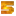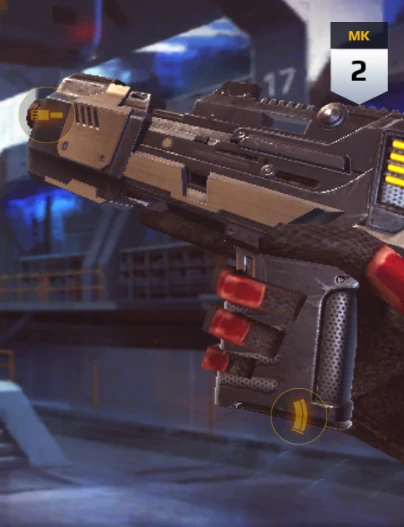The RAK-32 is a dual-wielded handgun featured in Modern Combat 5: Blackout.

## Modern Combat 5: Blackout

 RVK‑09 RAK‑32 RUN‑91 Prok Tonn Rokk Zekk Hans&Gret Cas&Poll

{{#infoboxbuilder: | 0:Theme = MC5 default | 0:CustomModule = | 0:MainImageCaption = | 0:ToggleContentLongerThan = 1500

| 1:Type = Title

```| 1:Value = RAK-32
```

| 2:Type = MainImage

```| 2:Value =```

| 3:Type = Line

```| 3:Label = Type
| 3:Value = Handgun
```

| 4:Type = Line

```| 4:Label = Likely based on
| 4:Value =
```

| 5:Type = Line

```| 5:Label = Users
| 5:Value =
```

| 6:Type = Line

```| 6:Label = Manufacturer
| 6:Value =
```

| 7:Value =

Statistics

| 8:Type = Line

```| 8:Label = Damage
| 8:Value = Above-Average
```

| 9:Type = Line

```| 9:Label = Radius
| 9:Value =
```

| 10:Type = Line

```| 10:Label = Rate of fire
| 10:Value = Extremely High
```

| 11:Type = Line

```| 11:Label = Range
| 11:Value = Very low
```

| 12:Type = Line

```| 12:Label = Accuracy
| 12:Value = Above-Average
```

| 13:Type = Line

```| 13:Label = Handling
| 13:Value =
```

| 14:Type = Line

```| 14:Label = Mobility
| 14:Value = Very High
```

| 15:Type = Line

```| 15:Label = Magazine size
| 15:Value = 15-16-17 (per weapon); 30-32-34 (combined)
```

| 16:Type = Line

```| 16:Label = Starting ammo
| 16:Value =
```

| 17:Type = Line

```| 17:Label = Max. ammo
| 17:Value =
```

| 18:Type = Line

```| 18:Label = Ammo received via pickup
| 18:Value =
```

| 19:Type = Line

```| 19:Label = Reloading
| 19:Value =
```

| 20:Type = Line

```| 20:Label = Fire mode
| 20:Value = Automatic
```

| 21:Type = Line

```| 21:Label = Effect
| 21:Value =

```

| 22:Type = Line

```| 22:Label = Max Capacity
| 22:Value =
```

| 23:Type = Line

```| 23:Label = Stealth
| 23:Value =
```

| 24:Type = Line

```| 24:Label = Countered by
| 24:Value =
```

| 25:Value =

Availability

| 26:Type = Line

```| 26:Label = Class
| 26:Value = Marauder
```

| 27:Type = Line

```| 27:Label = Tier
| 27:Value = 2
```

| 28:Type = Line

```| 28:Label = Unlocked
| 28:Value =
```

| 29:Type = Line

```| 29:Label = Cost
| 29:Value =
```

| 30:Type = Image

```| 30:Value =```

| 31:Type = Footer

```| 31:Value =
```

}}

The RAK-32 is a T2 dual-wielded handgun of the Marauder class. The weapon features notably better accuracy and higher mobility than comparable SMGs, but suffers from a noticeably lower rate of fire as its trade-off.

 + Solid accuracy High mobility Extremely high rate of fire − Fairly low ammo capacity